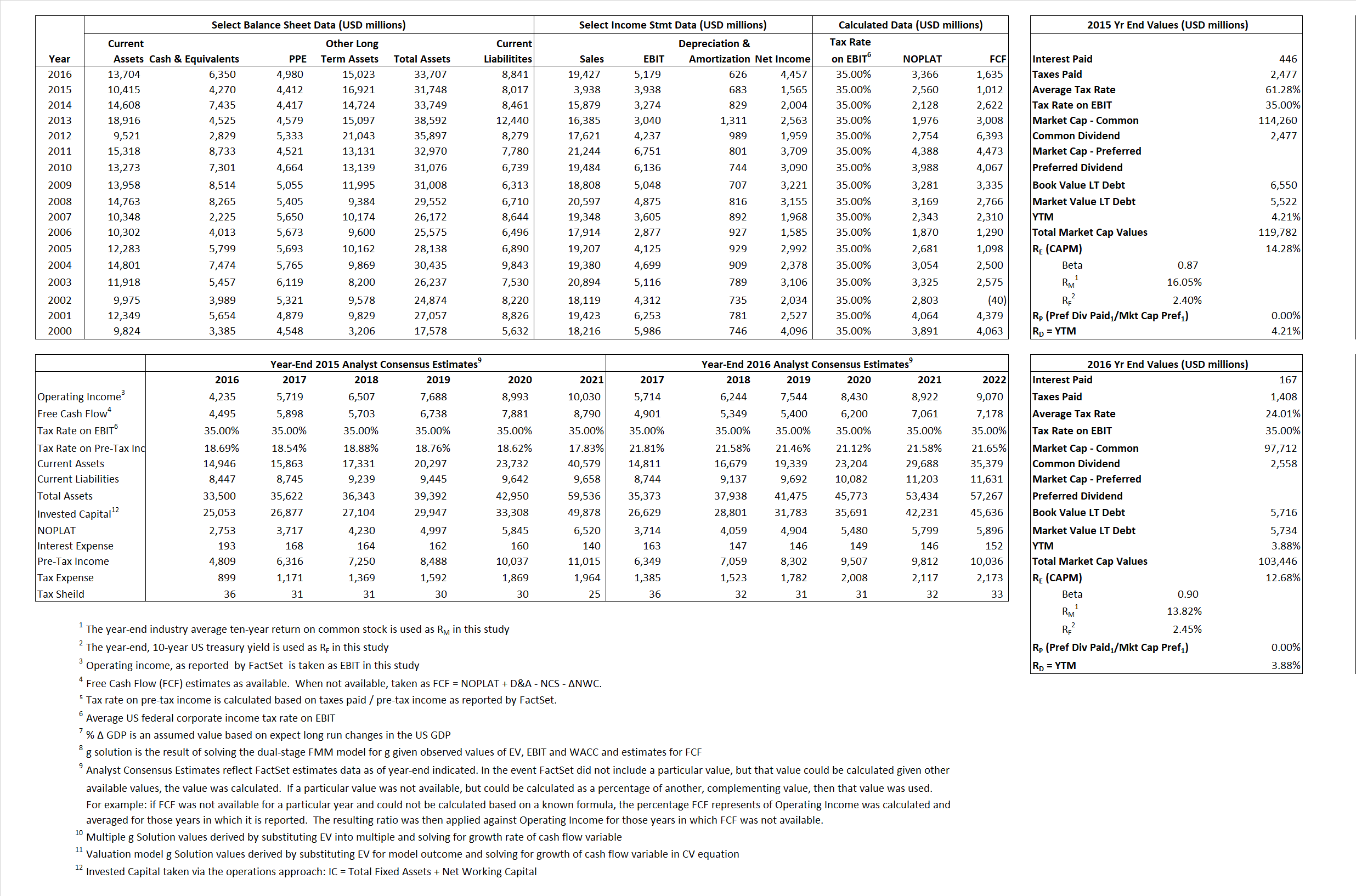# Bristol-Myers Squibb

## Analyst Listing

The following analysts provide coverage for the subject firm as of May 2016:

 Broker Analyst Analyst Email BMO Capital Markets Alex Arfaei alex.arfaei@bmo.com Deutsche Bank Research Gregg Gilbert gregg.gilbert@db.com William Blair John Sonnier jsonnier@williamblair.com SunTrust Robinson Humphrey John T. Boris john.t.boris@suntrust.com Piper Jaffray Richard J. Purkiss richard.j.purkiss@pjc.com Leerink Partners Seamus Fernandez seamus.fernandez@leerink.com Atlantic Equities Steve Chesney s.chesney@atlantic-equities.com Cowen & Company Steve Scala steve.scala@cowen.com Bernstein Research Tim Anderson tim.anderson@bernstein.com Guggenheim Securities Tony Butler tony.butler@guggenheimpartners.com Credit Suisse Vamil Divan vamil.divan@credit-suisse.com

## Primary Input Data## Derived Input Data

### Equational Form

Net Operating Profit Less Adjusted Taxes NOPLAT  2,560 3,366$NOPLAT\, =\, EBIT\, x\, (1 \,-\, Avg \,\,Tax\,\, Rate\,\, on\,\, EBIT)$
Free Cash Flow FCF 1,012 1,635$FCF\,=NOPLAT\,+\,Non-Cash\,Expenses-\Delta NWC\,-\,NCS$
Tax Shield TS 273 40$TS\,=\,Interest\,\,Paid\,\,x\,\, Avg \,\,Tax\,\,Rate\,\, on\,\, Pre-Tax\,\, Income$
Invested Capital IC 23,731 24,866$IC\,=\,Fixed\,\,Operating\,\,Assets\,\,+\,\,Net\,\, Working\,\, Capital$
Return on Invested Capital ROIC 10.79% 13.54%$ROIC\,=\,\frac { NOPLAT }{ IC }$
Net Investment NetInv (874)  1,761$NetInv\,=\,{ {IC}_{1}}-{{IC}_{0}}+Depreciation$
Investment Rate IR -34.14%  52.31%$IR\,=\,\frac {NetInv}{NOPLAT}$
Weighted Average Cost of Capital
WACCMarket  13.69% 12.14%$WACC\,=\,\frac { E }{ V } { R }_{ E }\,+\,\frac { P }{ V } { R }_{ P }\,+\,\frac { D }{ V } { R }_{ D }\left( 1- Avg\,\, Tax\,\,Rate\,\,on\,\,Pre-Tax\,\,Income \right)$
WACCBook    8.14%   7.89%
Enterprise value
EVMarket 115,512  97,096$EV\,=\,Market\,\,Cap\,\,Equity\,+\,\,Long\,\,Term\,\,Debt\,-\,Cash$
EVBook  116,540   97,078
Long-Run Growth
g = IR x ROIC
-3.68%   7.08% Long-run growth rates of the income variable are used in the Continuing Value portion of the valuation models.
g = %$\Delta$ GDP    2.50%    2.50%
Margin from Operations M  100.00%  26.66%$M\,\,=\,\,\frac{EBIT}{SALES}$
Depreciation/Amortization Rate D   14.78%  10.78%$D\,\,=\,\,\frac{D+A}{EBITDA}$

## Valuation Multiple Outcomes

The outcomes presented in this study are the result of original input data, derived data, and synthesized inputs.

### model g solution

12/31/2015 12/31/2016 12/31/2015 12/31/2016 12/31/2015 12/31/2016

EV/SALES$\frac {EV}{Sales} \,= \,\frac{ROIC\, -\, g}{ROIC\,(WACC\,-\,g)}\,(1\,-\,T)\,(M)$

29.33  5.00  14.44%  11.66%  14.13%  11.91%

EV/EBITDA$\frac {EV}{EBITDA} \,= \,\frac{ROIC\, -\, g}{ROIC\,(WACC\,-\,g)}\,(1\,-\,T)\,(1\,-\,D)$

25.00 16.73 14.44% 11.66% 14.13% 11.91%

EV/NOPLAT$\frac {EV}{NOPLAT} \,= \,\frac{ROIC\, -\, g}{ROIC\,(WACC\,-\,g)}$

45.13 28.84 14.44% 11.66% 14.13% 11.91%

EV/FCFOPS$\frac {EV}{FCF_{OPS}} \,= \,\frac{ROIC\, -\, g}{ROIC\,(WACC\,-\,g)}\,(1\,-\,T)$

114.14 59.39 14.44% 11.66% 14.13% 11.91%

EV/EBIT$\frac {EV}{EBIT} \,= \,\frac{ROIC\, -\, g}{ROIC\,(WACC\,-\,g)}\,(1\,-\,T)$

29.33 18.75 14.44% 11.66% 14.13% 11.91%

EV/IC$\frac {EV}{IC} \,= \,\frac{ROIC\, -\, g}{WACC\,-\,g}$

4.87 3.90 14.44% 11.66% 14.13% 11.91%